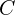Parameters in general are weights that are learned during training. They are weight matrices that contribute to the model’s predictive power, changed during the back-propagation process. The training algorithm and the optimization strategy make them change their values.

In a CNN, each layer has two kinds of parameters: weights and biases. The total number of parameters is just the sum of all weights and biases.

In this post, we share some formulas for calculating the number of parameters in a layer in a Convolutional Neural Network (CNN). This post does not define basic terminology used in a CNN and assumes you are familiar with them.

We will show the calculations using the Sequential model as an example. So, here is the PyTorch code for reference. Input: Color images of size `3x32x32`.

```model = nn.Sequential(
nn.ReLU(),
nn.ReLU(),
nn.MaxPool2d(2, 2), # output: 64 x 16 x 16
nn.BatchNorm2d(64),

nn.ReLU(),
nn.ReLU(),
nn.MaxPool2d(2, 2), # output: 128 x 8 x 8
nn.BatchNorm2d(128),

nn.ReLU(),
nn.ReLU(),
nn.MaxPool2d(2, 2), # output: 256 x 4 x 4
nn.BatchNorm2d(256),

nn.Flatten(),
nn.Linear(256*4*4, 1024),
nn.ReLU(),
nn.Linear(1024, 512),
nn.ReLU(),
nn.Linear(512, 10))
```

Input layer: The input layer has nothing to learn, it provides the input image’s shape. So no learnable parameters here. Thus the number of parameters = 0.

CONV layer: It has weight matrices and bias. To calculate the learnable parameters here, all we have to do is just multiply by the shape of width w, height h, previous layer’s filters d, and filters k in the current layer. Don’t forget the bias term for each of the filters. The number of parameters in a CONV layer would be : ((w * h * d)+1)* k), added 1 because of the bias term for each filter.

In Our model, at the first Conv Layer, the number of channels () of the input image is 3, the kernel size (WxH) is 3×3, and the number of kernels (K) is 32. So the number of parameters is given by:

``(((3x3x3)+1)*32)=896``

### Maxpooling2d Layers

The number of parameters for all MaxPooling2D layers is 0. The reason is that this layer doesn’t learn anything. What it does is reduce the complexity of the model and extract local features by finding the maximum values for each 2 x 2 pool.

### Fully Connected Layer (FC):

In comparison to the other layers, this layer has the highest number of parameters, because, every neuron is connected to every other neuron. It is the product of the number of neurons in the current layer c and the number of neurons in the previous layer p and as always, do not forget the bias term. Thus a number of parameters here are: ((current layer neurons c * previous layer neurons p)+1*c).

The total number of parameters in Our model is the sum of all parameters in the 6 Conv Layers + 3 FC Layers. It comes out to a whopping 5,852,234. The table below provides a summary.

The summary() function will create a summary for the model. Each row represents a layer with each named uniquely such that we can refer to these layers without any ambiguity.

Each layer has an output and its shape is shown in the “Output Shape” column. Each layer’s output becomes the input for the subsequent layer. The “Param #” column shows you the number of parameters that are trained for each layer.

The total number of parameters is shown at the end, which is equal to the number of trainable and non-trainable parameters. In this model, all the layers are trainable.

`trainable_weights` is the list of those that are meant to be updated via gradient descent to minimize the loss during training.`non_trainable_weights` is the list of those that aren’t meant to be trained. Typically they are updated by the model during the forward pass.